# Valko - McCain bubble point pressure correlation

Jump to: navigation, search

## Valko - McCain bubble point pressure correlation

Valko - McCain is an empirical correlation for the bubble point pressure published in 2003 .

## Math & Physics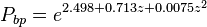$P_{bp} = e^{2.498 + 0.713 z + 0.0075 z^2}$

where: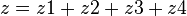$z = z1 + z2 + z3 + z4$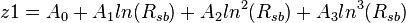$z1 = A_0+ A_1 ln(R_{sb}) + A_2 ln^2(R_{sb}) + A_3 ln^3(R_{sb})$

A0 = -4.814074834
A1 = 0.7480913
A2 = 0.1743556
A3 = -0.0206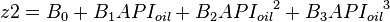$z2 = B_0 + B_1 API_{oil} + B_2 {API_{oil}}^2 + B_3 {API_{oil}}^3$

B0 = 1.27
B1 = -0.0449
B2 = 4.36e-4
B3 = -4.76e-6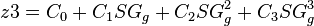$z3 = C_0 + C_1 SG_{g} + C_2 SG^2_{g} + C_3 SG^3_{g}$

C0 = 4.51
C1 = -10.84
C2 = 8.39
C3 = -2.34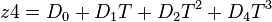$z4 = D_0 + D_1 T + D_2 T^2 + D_4 T^3$

D0 = -7.2254661
D1 = 0.043155
D2 = -8.55e-5
D3 = 6.00696e-8

## Application range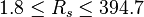$1.8 \le R_s \le 394.7$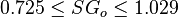$0.725 \le SG_o \le 1.029$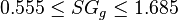$0.555 \le SG_g \le 1.685$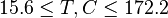$15.6 \le T, C \le 172.2$

## Example. Calculation of the bubble point pressure

Example source 

### Input data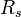$R_s$ = 60 sm3/sm3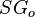$SG_o$ = 0.85 or 35 API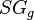$SG_g$ = 0.75$T$ = 90C or 363K

Calculate bubble point pressure?

### Solution

z1=-0.2422
z2=0.0294
z3=0.1122
z4=-0.0594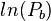$ln(P_b)$ = 2.4557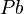$Pb$ = 11.65MPa or 115 atm

The solution is available in the online PVT calculator software at www.pengtools.com

## Nomenclature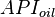$API_{oil}$ = oil specific gravity, °API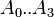$A_0..A_3$ = coefficients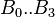$B_0..B_3$ = coefficients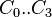$C_0..C_3$ = coefficients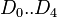$D_0..D_4$ = coefficients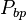$P_{bp}$ = bubble point pressure, MPa$R_s$ = solution oil gas ratio, sm3/sm3$SG_g$ = gas specific gravity, dimensionless$SG_o$ = oil specific gravity, dimensionless$T$ = temperature, °K